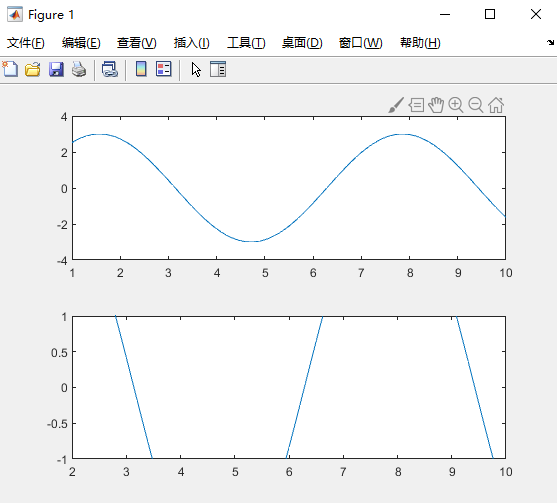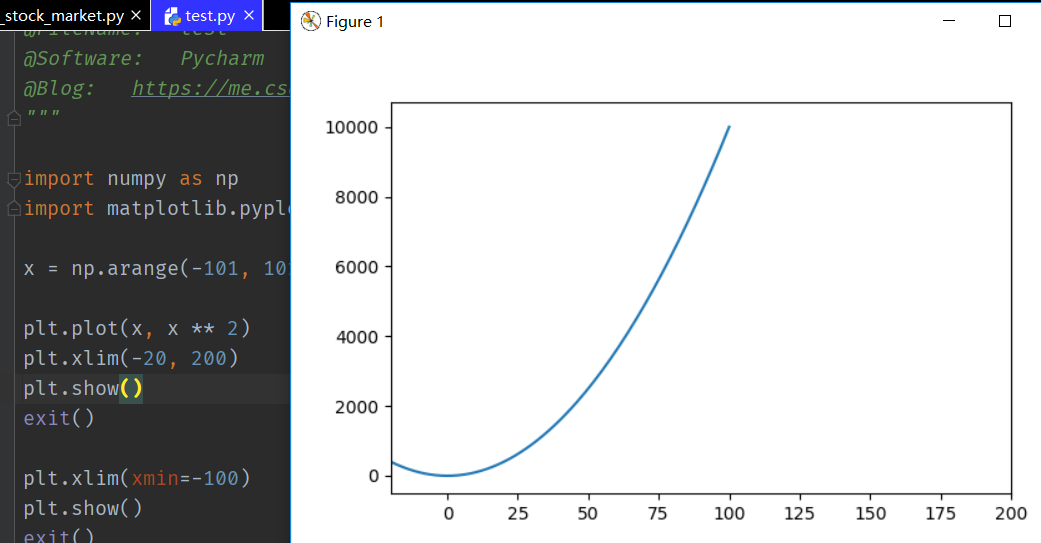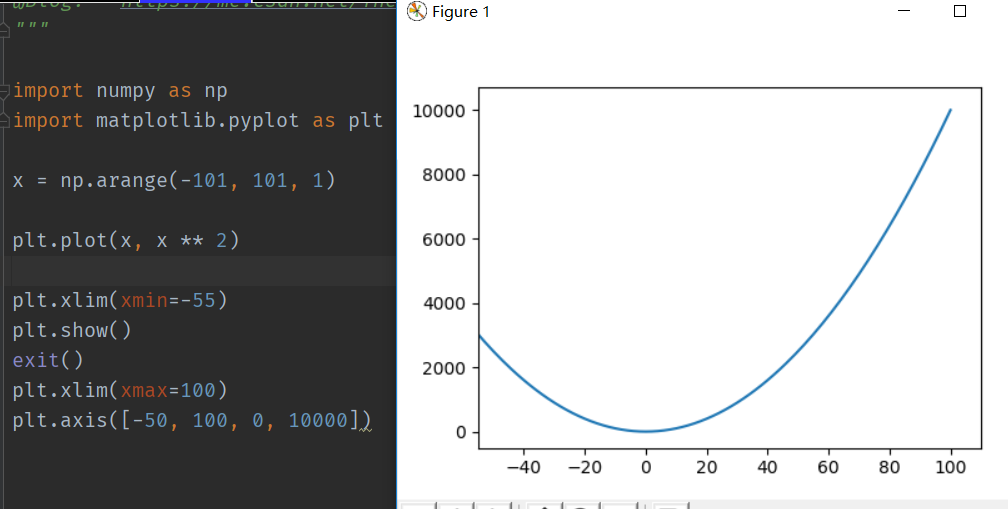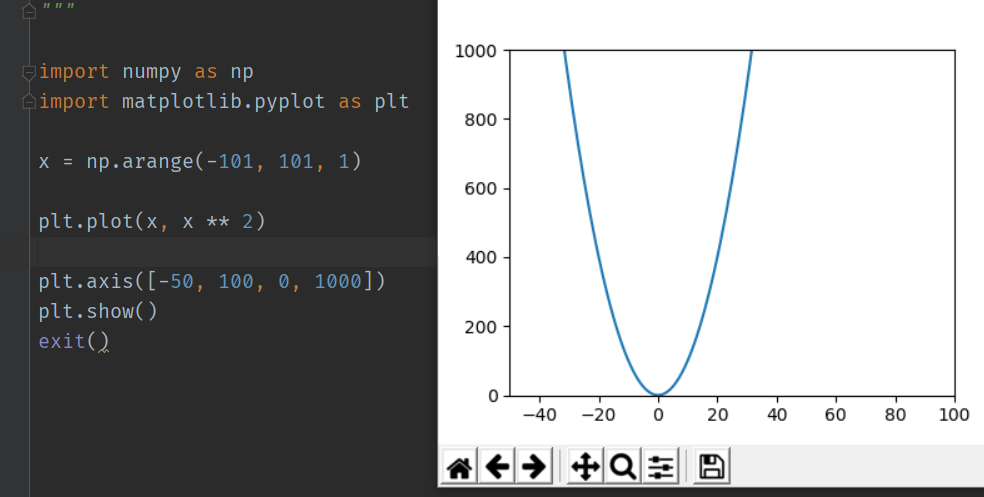• 设置 xlim（或缩放）后，有时将 ylim 设置为 ydata WITHIN xlim 的全范围很有用。 ylim([-inf inf]) 将在 min(ydata) 的 ylim 设置为 max(ydata)。“轴紧”也是如此，并且还设置了 xlim([min(xdata) max(xdata)])。 ...matlab
• 设置 xlim 后，有时将 ylim 设置为 xlim 内的 ydata 范围很有用。 “轴紧”会将 xlim 和 ylim 设置为完整 xdata 和 ydata 的范围。 setytight 将不影响 xlim，并将 ylim 设置为 xlim 内的 ydata 范围。 例如： 情节...matlab
• 由于至少包含在 R12 和 R13 中，函数 XLIM、YLIM 和 ZLIM 将无法正确编译。 该文件包含这些文件的修改版本，编译时不会产生错误。 将此文件的内容解压缩到您的 \$MATLABroot\toolbox\matlab\graph3d\ 目录。matlab
• xlim函数使用–Matplotlib **函数功能：**Get or set the x limits of the current axes        获取或设置x轴数值显示范围 函数语法： left, right = xlim() # return the current xlim 返回当前x轴边界 xlim...
xlim函数使用–Matplotlib
xlim 语法、功能与参数设置可平移至ylim
**函数功能：**Get or set the x limits of the current axes        获取或设置x轴数值显示范围 函数语法：
left, right = xlim()  # return the current xlim   返回当前x轴边界
xlim((left, right))   # set the xlim to left, right  设置x轴边界
xlim(left, right)     # set the xlim to left, right   设置x轴边界

函数参数： left: xmin，x轴的最小值 right: xmax, x轴的最大值
获取x轴数值显示范围：plt.xlim()
下图中，x轴的最小值为-0.4475，最大值为10.4975调整x轴边界，最小值为0，最大值为10第一次出现的plt.xlim()，因为plt.xlim()函数在x轴调整边界前面，其输出结果时尚未对x轴边界进行重新定义，输出值依然为默认值，而非修改后的值。 第二次出现的plt.xlim()，图形x轴已经函数调整边界，其输出结果为修改后的值。 设置y轴边界，以输入双括号的形式，可以看到与x使用的单括号并无差别。当同时调整左右边界时可以使用位置传参，还可以只调整单侧边界，使用关键字传参，此时只能使用单括号一种形式 修改x轴的最大值
xlim(right=3)  # adjust the right leaving left unchanged   只调整右侧边界
xlim(left=1)  # adjust the left leaving right unchanged    只调整左侧边界展开全文python
• xlim([xl xr]); 用于限定x轴上下限，其中， xl：x轴下限 xr：x轴上限 ylim([yl yr]);zlim([yl yr])同理。 例子 x = [1:0.1:10]; y = 3*sin(x); subplot(2,1,1); plot(x,y) subplot(2,1,2); plot(x,y)； ylim([-1,...
xlim([xl xr]);  用于限定x轴上下限，其中，  xl：x轴下限  xr：x轴上限  ylim([yl yr]);zlim([yl yr])同理。
例子
x = [1:0.1:10];
y = 3*sin(x);
subplot(2,1,1);
plot(x,y)
subplot(2,1,2);
plot(x,y)；
ylim([-1,1]);想了解更多参考【 MATLAB 】xlim 、 ylim 、zlim简介
展开全文Matlab zlim
• ## Matlab xlim ylim函数

万次阅读 多人点赞 2017-07-31 15:11:39
xlim([xl xr]); ylim([yl yr]); 用于限定x轴 y轴上线限制，其中， xl：x轴下限 xr：x轴上限 yl：y轴下限 yr：y轴上限
xlim([xl xr]);  ylim([yl yr]);  用于限定x轴 y轴上线限制，其中，  xl：x轴下限  xr：x轴上限  yl：y轴下限  yr：y轴上限
展开全文• matplotlib.pyplot.xlim() 官方文档 matplotlib.pyplot.xlim(*args, **kwargs) 获取或者是设定x座标轴的范围，当前axes上的座标轴。 有两种参数输入方式 plt.xlim(num1, num2) plt.xlim(xmin=num1,xmax=...
 matplotlib.pyplot.xlim() 官方文档
matplotlib.pyplot.xlim(*args, **kwargs)

获取或者是设定x座标轴的范围，当前axes上的座标轴。
有两种参数输入方式
plt.xlim(num1, num2)plt.xlim(xmin=num1,xmax=num2)matplotlib.pyplot.ylim 官方文档
内容与x一样
matplotlib.pyplot.axis 官方文档
matplotlib.pyplot.axis(*v, **kwargs)

参数详解：
xmin, xmax, ymin, ymax : float, optional  option : str
ValueDescription‘on’Turn on axis lines and labels.‘off’Turn off axis lines and labels.‘equal’Set equal scaling (i.e., make circles circular) by changing axis limits.‘scaled’Set equal scaling (i.e., make circles circular) by changing dimensions of the plot box.‘tight’Set limits just large enough to show all data.‘auto’Automatic scaling (fill plot box with data).‘normal’Same as ‘auto’; deprecated.‘image’‘scaled’ with axis limits equal to data limits.‘square’Square plot; similar to ‘scaled’, but initially forcing xmax-xmin = ymax-ymin. emit : bool, optional, default True展开全文• pyplot模块中的autoscale函数可以切换是否自动缩放坐标轴范围，xlim()和ylim()函数可手动设置坐标轴范围。 autoscale函数 对于pyplot模块控制坐标轴范围是否自动缩放的函数为autoscale。 函数签名为matplotlib....matplotlib 坐标轴范围
• ## matplotlib中的set_xlim

千次阅读 2020-06-11 21:17:00
···python plt.title("Model with Adam optimization") ...axes.set_xlim([-1.5,2.5]) axes.set_ylim([-1,1.5]) plot_decision_boundary(lambda x: predict_dec(parameters, x.T), train_X, trai...
• xlim()与ylim()–设置数值显示范围 函数格式： plt.xlim(xmin,xmax) plt.ylim(ymin,ymax) 参数说明： xmin：x轴上的显示下限 xmax：x轴上的显示上限 代码： import matplotlib.pyplot as plt import numpy as np ...python 数据可视化
• fix_x_limits 将当前图形中的所有轴固定到找到的最大跨度fix_x_limits(h) 修复 h 中所有轴对象或 h 下所有轴的 XLim 属性，如果图形处理到所有轴中找到的最大跨度fix_x_limits(h,limits) 修复所有给定的限制：[minx ...matlab
• datenow = datetime.datetime.now dstart = datetime(2015,4,1) print datenow plt.ylim(0, .14) plt.xlim(dstart, datenow) The xlim is what I am struggling with. I'm getting the error File "C:/Mypath/name....
• 书上的图是这样：而直接运行该页程序得到的图是这样：这时候需要加上设定x轴范围的语句：plt.xlim(dates , dates[-1] )。整个程序如下：import csvimport matplotlib.pyplot as pltfrom datetime import da...
• 调用签名：plt.xlim(xmin, xmax) xmin：x轴上的最小值 xmax：x轴上的最大值 平移性：上面的函数功能，调用签名和参数说明同样可以平移到函数ylim()上。 代码实现： import matplotlib.pyplot as plt import ...
• matplotlib-03 xlim设置x轴的显示范围功能介绍代码展示重点代码解释 功能介绍 对竖轴的显示范围进行设置，可以实现对画出的图进行裁剪或者移动的操作。综合的对x轴和y轴的显示范围进行控制，在保证图幅大小不变时，...可视化 数据可视化 python
• xlim Set or query x-axis limits Syntax xlim(limits) xl = xlim xlim auto xlim manual m = xlim('mode') ___ = xlim(target,___) Description xlim(limits) sets the x-axis limits for the current axes or...
• 数据分析xlim().ylim()函数样例展示 xlim().ylim()函数 函数功能：设置x轴/y轴的数值显示范围。 调用签名：plt.xlim(xmin, xmax) / plt.ylim(ymin, ymax) xmin：x轴上的最小值 xmax：x轴上的最大值 平移性...python 数据可视化
• import matplotlib.pyplot as plt import numpy as np x=np.linspace(0.05,10,1000) y=np.random.rand(1000) plt.scatter(x,y,label="scatter figure") ...plt.xlim(0.05,10) plt.ylim(0,1) plt.show...
• import matplotlib.pyplot as plt import numpy as np x = np.linspace(0.05,10,1000) y = np.random.rand(1000) plt.scatter(x,y,label="scatter figure") ...plt.xlim(1,10) plt.ylim(0,1) plt.show()
• 错误使用 xlim (line 31) 范围必须为包含递增的日期时间值的 2 元素向量。 具体图片： 出现这样问题的原因是我想以日期为x轴，数据为y轴，并且约束一下坐标轴范围。因为日期是datetime类型，所以我用了xlim来约束x...matlab
• import matplotlib.pyplot as plt import numpy as np x = np.linspace(-1,1,5...plt.xlim((-1,6)) new_ticks = np.linspace(-1,2,5) plt.xticks(new_ticks) plt.plot(x,y,'ob-') plt.show() 改变xticks——坐标轴...
• import matplotlib.pyplot as plt import numpy as np #从[-1,1]中等距去50个数作为x的取值 x=np.linspace(-1,1,5)#图像仅在-1到1...plt.xlim((-1,5)) #设置点的位置 new_ticks = np.linspace(-1,2,5) plt.xtick...
• get_xlim返回当前Axes视图的x的上下限, get_ylim同. 如: >>>print('xlim:\n',xlim,'\n') >>>print('xlim的类型:\n',type(xlim),'\n') xlim: (0.0, 1.0) xlim的类型: <class 'tuple'> ...
• 1.画图代码： import matplotlib.pyplot as plt import numpy as np #从[-1,1]中等距去50个数作为x的取值 x=np.linspace(-1,1,5)#...plt.xlim((-1,5)) 设置点的位置 x轴的刻度显示在-1到2之间 new_ticks = np....
• I'm trying to get the xlimits of a plot as a python datetime object from a time series plot created with ... Using ax.get_xlim() returns the axis limits as a numpy.float64, and I can't figure out...
• 不设置上下限，曲线都跑出关注区域了。。。 参考文章：matplotlib 设定坐标的上下限：matplotlib.pyplot.xlim
• 目录1、set_xlim()、set_ylim()2、 xticks() 、yticks()、set_xlabels()、set_ylabels()3、双坐标轴 1、set_xlim()、set_ylim() Matplotlib会自动得出沿x、y（和z轴，如果是3D图）轴显示的变量的最小值和最大值。...
• 使用xlim(x1,x2) 设定横坐标范围, 使用ylim(y1,y2) 设定纵坐标范围 import numpy as np import matplotlib.pyplot as plt x=np.arange(-5,5,0.1) y=x**2 plt.xlim(-5,5) #设定横坐标范围 plt.ylim(0,100) #...python
• 看两段代码，亲 import matplotlib.pyplot as plt import numpy as np t = [1,2,3,4,5] ...ax.set_xlim(0, 10) ax.set_ylim(0, 10) plt.show() 结果： import matplotlib.pyplot as plt import...matplotlib python 数据可视化
•python...